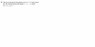# Finding the equation of a plane

Miike012
The question is in the paint doc.

My solution:
I created a matrix

1. first row: 1,1,-1|2 which represents the equation of the plane x + y - z = 2
second row: 2,-1,3|1 ... 2x - y + 3z = 1

2. I came up with the parametric equation:

x = 1 -2/3t
y = 1 + 5/3t
z=t

3. The vector that represents this line is:
<-2/3,5/3,0> = <-2,5,0>

4. A point on the line is (1,1,0)

5. <-1,2,1> - <1,1,0> = <-2,1,1> ... Vector // to plane Note: (-1,2,1) is a point on the plane.

6. <-2,1,1> x <-2,5,0> = <5,2,8>

7. 5(x-1) + 2(y-1) + z = 0
or
5x + 2y + z = 7

The answer in the back of the book is: x - 2y + 4z = -1

#### Attachments

•plane.jpg
4.1 KB · Views: 377